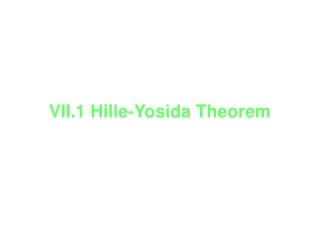Download PresentationVII.1 Hille-Yosida Theorem

# VII.1 Hille-Yosida Theorem - PowerPoint PPT PresentationDownload Presentation## VII.1 Hille-Yosida Theorem

- - - - - - - - - - - - - - - - - - - - - - - - - - - E N D - - - - - - - - - - - - - - - - - - - - - - - - - - -
##### Presentation Transcript

1. VII.1 Hille-Yosida Theorem

2. VII.1 Definition and Elementary Properties of maximal monotone operators

3. Maximal Monotone Let H be a real Hilbert space and let be an unbounded linear operator . A is called monotone if A is called maximal monotone if furthermore i.e.

4. Proposition VII.1 Let A be maximal monotone. Then (a) D(A) is dense in H (b) A is closed. (c) For every is a bijection from D(A) onto H is a bounded operator with

5. Yosida Regularization of A Let A be maximal monotone, for each let (by Prop.VII.1 ) is called a resolvent of A and is called Yosida regularization of A

6. Proposition VII. 2 p.1 Let A be maximal monotone, Then (a1) (a2) (b) (c)

7. Proposition VII. 2 p.2 (d) (e) (f)

8. VII.2 Solution of problem of evolution

9. Theorem VII.3 Cauchy, Lipschitz. Picard Let E be a Banach space and F be a mapping From E to E such that there is a unique then for all such that

10. Lemma VII.1 If is a function satisfing , then the functions and are decreasing on

11. Theorem VII.4 (Hille-Yosida) p.1 Let A be a maximal monotone operator in a Hilbert space H then for all there is a unique s.t.

12. Theorem VII.4(Hille-Yosida) where D(A) is equipped with graph norm i.e. for Furthermore, and

13. Lemma VI.1 (Riesz-Lemma) Let For any fixed , apply Green’s second identity to u and in the domain we have and then let